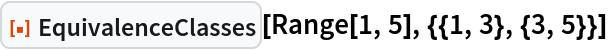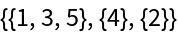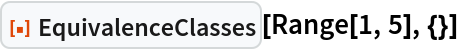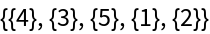Function Repository Resource:

# EquivalenceClasses

Compute the equivalence classes associated with a given set of elementwise identifications

Contributed by: Shuheng Li
 ResourceFunction["EquivalenceClasses"][list,{{a1,a2},{b1,b2},…}] partitions list into equivalence classes according to the relations a1~a2,b1~b2,….

## Details

The minimal equivalence relation containing the relations a1~a2, b1~b2,… is assumed.

## Examples

### Basic Examples

Equivalence classes of Range[1,5] under the relations 1~3 and 3~5:

 In:=Out=If no relations are given, EquivalenceClasses returns the singleton elements of list:

 In:=Out=Shuheng Li

## Version History

• 1.0.0 – 04 November 2022